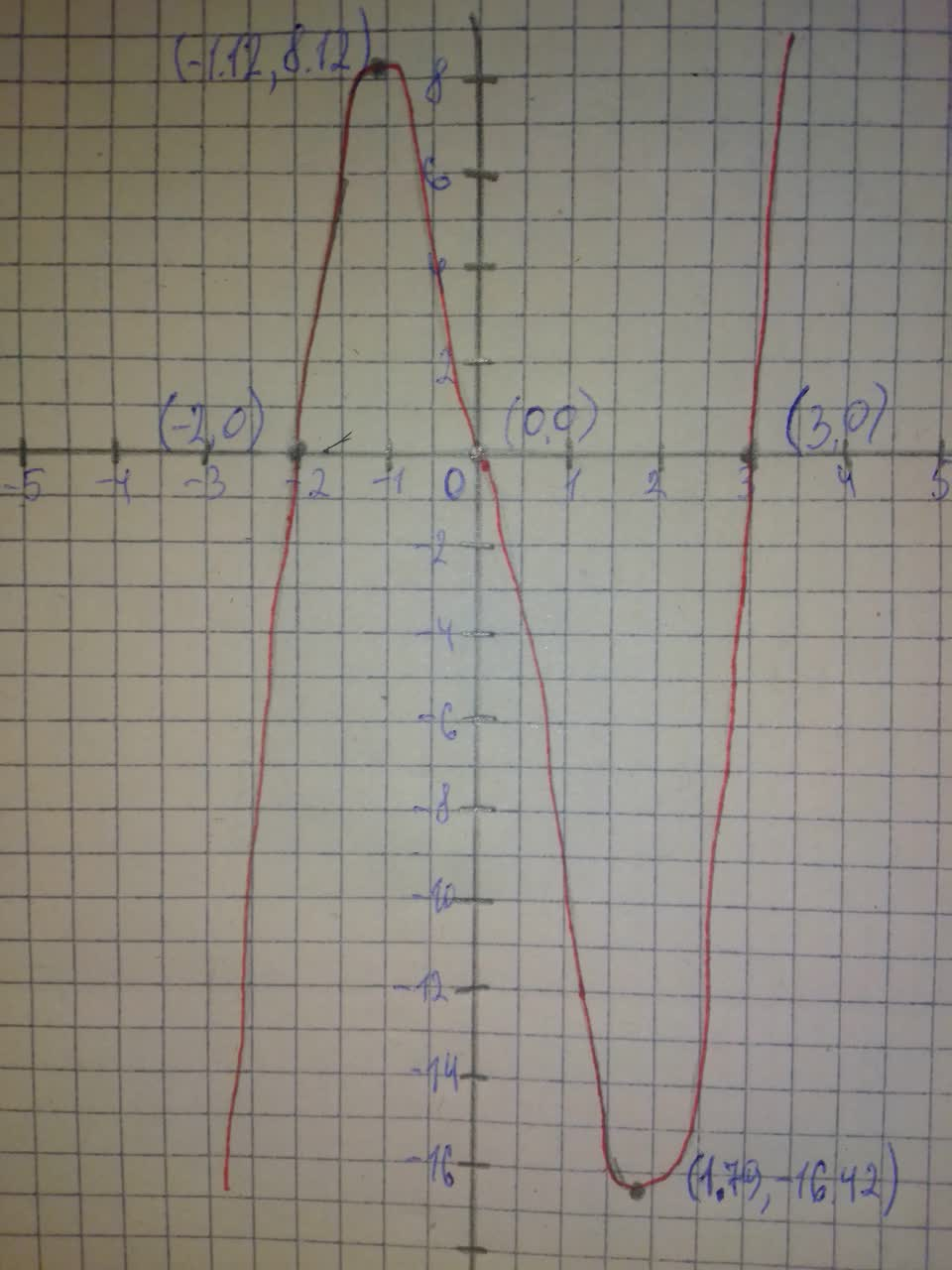Question# Graph each polynomial function. Factor first if the expression is

Polynomial graphs
ANSWEREDGraph each polynomial function. Factor first if the expression is not in factored form. $$\displaystyle{f{{\left({x}\right)}}}={2}{x}{\left({x}-{3}\right)}{\left({x}+{2}\right)}$$2021-08-17
Step 1
$$\displaystyle{f{{\left({x}\right)}}}={2}{x}{\left({x}-{3}\right)}{\left({x}+{2}\right)}$$ The function in factored form
The function has three zeros 0,3, and -2
So, the graph of f(x) crosses the x-axis at (0,0),(3,0), and (-2,0)
To find the y-intercept, substitute 0 for x in f(x)
$$\displaystyle{f{{\left({x}\right)}}}={2}{x}{\left({x}-{3}\right)}{\left({x}+{2}\right)}$$
$$\displaystyle{f{{\left({0}\right)}}}={2}{\left({0}\right)}{\left({0}-{3}\right)}{\left({0}+{2}\right)}$$ Substitute 0 for x
$$\displaystyle={0}$$
So, the function f(x) crosses the y-axis at (0,0)
Step 2
$$\displaystyle{2}{x}\cdot{x}\cdot{x}={2}{x}^{{{3}}}$$
$$\displaystyle{x}\rightarrow\infty,{f{{\left({x}\right)}}}\rightarrow\infty$$
$$\displaystyle{x}\rightarrow-\infty,{f{{\left({x}\right)}}}\rightarrow-\infty$$